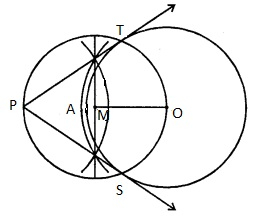# Draw two tangents to a circle of radius 3.5 cm from a point P at a distance of 6.2 cm from its centre.

Given:

A circle of radius 3.5 cm.

Point P is at a distance of 6.2 cm from its centre.

To do:

We have to draw two tangents to a circle of raidus 3.5 cm from a point P at a distance of 6.2 cm from its centre.

Solution:Steps of construction:

(i) Draw a circle with centre $O$ and radius $3.5\ cm$

(ii) Take a point $P$ which is $6.2\ cm$ from $O.$

(iii) Bisect $PO$ at $M$ and draw a circle with centre $M$ and diameter $OP$ which intersects the given circle at $T$ and $S$ respectively.

(iv) Join $PT$ and $PS$.

$PT$ and $PS$ are the required tangents to the circle.

Updated on: 10-Oct-2022

31 Views